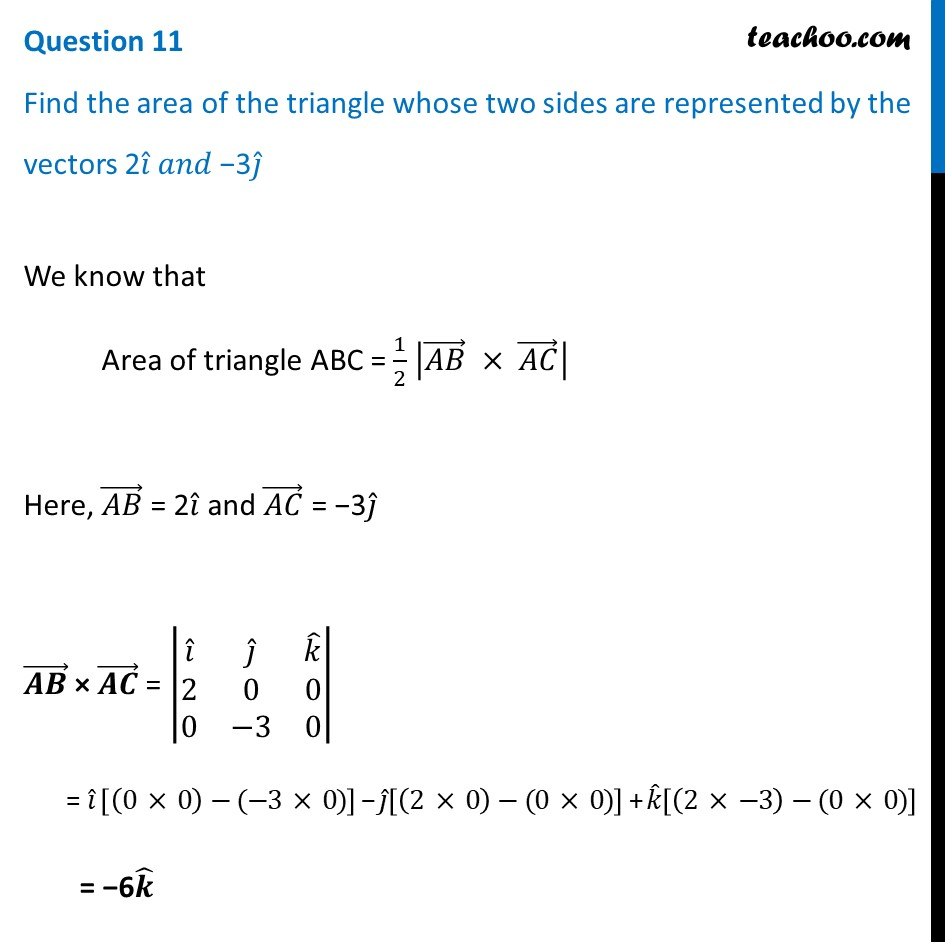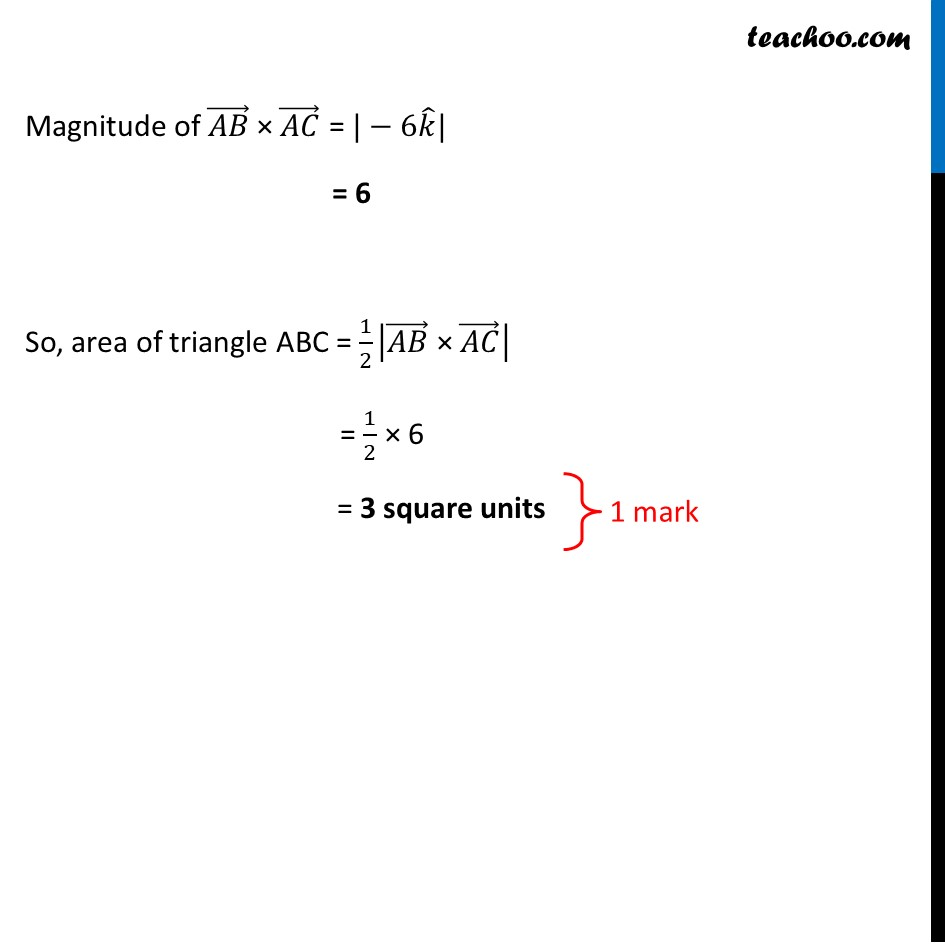## Find the area of the triangle whose two sides are represented by the vectors 2i ̂ 𝑎𝑛𝑑 − 3j ̂.1. Class 12
2. Solutions of Sample Papers and Past Year Papers - for Class 12 Boards
3. CBSE Class 12 Sample Paper for 2021 Boards

Transcript

Question 11 Find the area of the triangle whose two sides are represented by the vectors 2𝑖 ̂ 𝑎𝑛𝑑 −3𝑗 ̂ We know that Area of triangle ABC = 1/2 |(𝐴𝐵) ⃗ × (𝐴𝐶) ⃗ | Here, (𝐴𝐵) ⃗ = 2𝑖 ̂ and (𝐴𝐶) ⃗ = −3𝑗 ̂ (𝑨𝑩) ⃗ × (𝑨𝑪) ⃗ = |■8(𝑖 ̂&𝑗 ̂&𝑘 ̂@2&0&0@0&−3&0)| = 𝑖 ̂ [(0 × 0)−(−3 × 0)] − 𝑗 ̂[(2 × 0)−(0 × 0)] + 𝑘 ̂[(2 × −3)−(0 × 0)] = −6𝒌 ̂ Magnitude of (𝐴𝐵) ⃗ × (𝐴𝐶) ⃗ = |−6𝑘 ̂| = 6 So, area of triangle ABC = 1/2 |(𝐴𝐵) ⃗" × " (𝐴𝐶) ⃗ | = 1/2 × 6 = 3 square units

CBSE Class 12 Sample Paper for 2021 Boards

Class 12
Solutions of Sample Papers and Past Year Papers - for Class 12 Boards